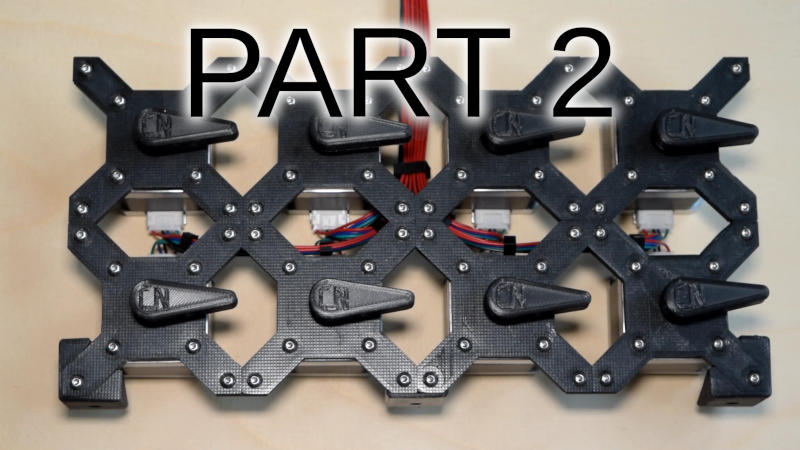# Play "Music" with stepper motors Part 2

The basic hardware was described in Play "Music" with stepper motors Part 1 this time I'm showing and explaining the software.
This part is a bit more tricky.

I've used the normal Arduino IDE for it, I'm not a big fan of it, but it gets the job done and most of the readers have it already set up.

The software can be downloaded here, I'll only copy/paste the relevant parts here.

The setup function is straight forward and boring, the only thing is that you can configure the number of channels (if you build less than 8) and the value is stored in EEPROM at position 0.
This is stored in a local variable.
The fun part is in the loop function:

`    Serial.readBytes(msg, 3);    uint8_t nibble = msg >> 4;`

Simple so far, read 3 bytes from serial port and store the highest 4 bit of the first byte.

Why? Simple: The midi format has the following data:
Byte 0: Message type (4 bits) Channel (4 bits)
Depending on message type, byte 1 and 2 contains some info about it.
We are only interested in 2 types, both have the midi note in byte 1 and byte 2 is the velocity, think of volume.
We ignore velocity, and just use the note. And a third type for turning off/reset.
So for tuning a note on, we get message 0b1001 and code for it starts with a quick check if we are already
powered on. If not, power up.

`    if (nibble == 0b1001) {  // note on      if (!power) { // not powered on yet?        // enable steppers        power = true;        m2s.power(true);      }`

The next part is to calculate the frequency from the midi note value.
The formular is taken from the midi spec, it is 2((midi value-69)/12) * 440Hz. About type conversion is needed
otherwise integer calculation is used, which is not what we want.

`      // calculate frequency from midi note      uint16_t freq = pow(2, (static_cast<double>(msg) - 69.0)/12.0) * 440.0;      // velocity byte 2 is ignored`

The next part is more an artistic choice, but I use an algorithm that utilizes all steppers by sending the next node
to the next free stepper, not just starting from one specific stepper.

`      // loop through channels, starting with nextChannel to find the next free one      uint8_t channel = nextChannel;      bool good = false;      do {        if (channels[channel] == 0) {          m2s.setChannel(channel, freq);          channels[channel] = freq;          nextChannel = (channel + 1) % (numChannels);  // next channel = current channel + 1 modulo num channels          good = true;          break;        }        channel = (channel + 1) % numChannels;      } while (channel != nextChannel);  // if we end at our start channel, no free channels, ignore note      if (!good && sendStatus) {        Serial.print(F("\ntoo many channels\n"));      }    }`

The next stepper to try is stored in nextChannel. The algorithm loops through all channels starting from that and when there is a free channel,
it will be used. nextChannel is update and we are done. If there are no channels free (more than 8 simultaneous notes) we just ignore it and optionally

the note off is a bit simpler:

`if (nibble == 0b1000) {  // note off      uint16_t freq = pow(2, (static_cast<double>(msg) - 69.0)/12.0) * 440.0;      // velocity byte 2 is ignored      // loop through channels to find an active one with that frequency      for (uint8_t channel = 0; channel < numChannels; ++channel) {        if (channels[channel] == freq) {          m2s.setChannel(channel, 0);          channels[channel] = 0;          break;        }      }    }`

It just looks for a channel with given frequency and turns that off.

And finally a control message check

`    if (nibble == 0b1011) {  // control      switch (msg) {        case 121:  // reset        case 123:  // all notes off          if (power) {            power = false;            m2s.power(false);          }          break;      }    }`

We only care about message 121 and 123 to turn off.

The ugly and difficult part is in hidden in the midi2stepper.cpp/.h this will be part 3.